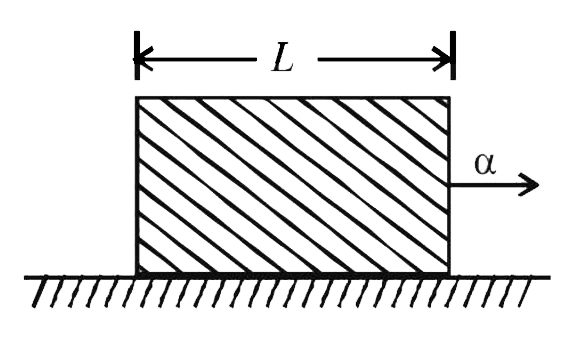# A uniform rod of length L and density rho is being pulled along a smooth floor with a horizontal acceleration alpha (see Fig.) The magnitude of th

28 views
in Physics
closed
A uniform rod of length L and density rho is being pulled along a smooth floor with a horizontal acceleration alpha (see Fig.) The magnitude of the stress at the transverse cross-section through the mid-point of the rod is……..by (90.1k points)
selected

Let A be the area of cross-section of the rod.
Consider the back half portion of the rod.
Mass of half portion of the rod =(rhoAL)/(2)
The force responsible for its acceleration is
f=(rhoAL)/(2)xxa :. Stress =f/A=(rhoLalpha)/(2)